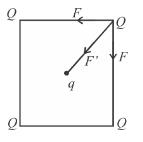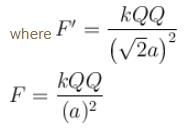Courses

# Electrostatics MCQ Level - 1

## 10 Questions MCQ Test Basic Physics for IIT JAM | Electrostatics MCQ Level - 1

Description
This mock test of Electrostatics MCQ Level - 1 for Physics helps you for every Physics entrance exam. This contains 10 Multiple Choice Questions for Physics Electrostatics MCQ Level - 1 (mcq) to study with solutions a complete question bank. The solved questions answers in this Electrostatics MCQ Level - 1 quiz give you a good mix of easy questions and tough questions. Physics students definitely take this Electrostatics MCQ Level - 1 exercise for a better result in the exam. You can find other Electrostatics MCQ Level - 1 extra questions, long questions & short questions for Physics on EduRev as well by searching above.
QUESTION: 1

### A particle of charge –q and mass m moves in a circle of radius r around an infinitely long line charge on linear charge density +λ. Then time period will be :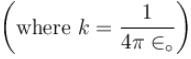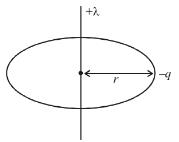Solution:

We have centripetal force equation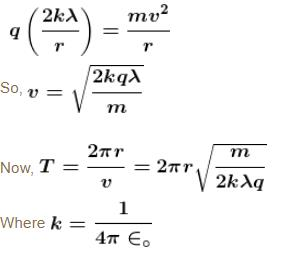The correct answer is: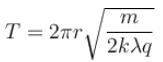QUESTION: 2

### Figure, shown above shows three situations involving a charged particle and a uniformly charged spherical shell. The charges and radii of the shells are indicated in the figure. If F1, F2 and F3 are the magnitudes of the force on the particle due to the presence of the shell in situations (I), (II) and (III) respectively then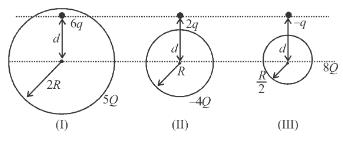Solution:

F1 is zero as charges lies inside the shell (Electric field inside the shell due to its own charge is zero). F2 = F3  as charge over the surface of the shell behaves like concentrated at the centre.
The correct answer is: F3 = F2 > F1

QUESTION: 3

### There are three concentric thin spheres of radius a, b, c (a > b > c).  The total surface charge densities on their surfaces are σ, - σ, σ respectively. The magnitude of electric field at r (distance from centre) such that  a > r > b  is

Solution:

Electric field at a distance r (a > r > b)  will be due to charges enclosed in r only, and since, a sphere acts as a point charge for points outside its

surface.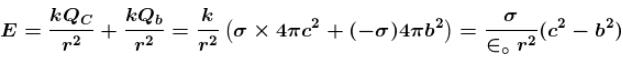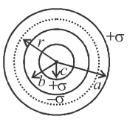The correct answer is: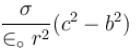QUESTION: 4

A point charge Q is located at centre of a fixed thin ring of radius R with uniformly distributed charge –Q. The magnitude of the electric field strength at the point lying on the axis of the ring at a distance x from the centre is
(x >> R)

Solution: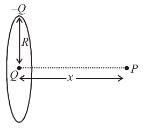Electric field due to uniformly charged ring at its’ exist (at distance x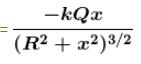and Electric field due to point charge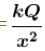So net electric field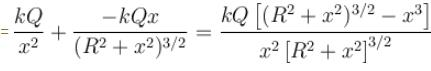When R < < x,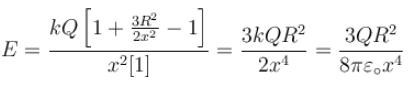The correct answer is: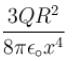QUESTION: 5

A charged particle q is shot towards another charged particle Q which is fixed, with a speed v. It approaches Q upto a closest distance r and then returns. If q was given a speed 2v, the closest distance of approach would be :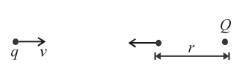Solution:

From the given data, using energy conservation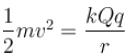When particle is shot with a speed 2v.

Let distance of closest approach = x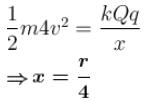QUESTION: 6

Two semicircular rings lying in same plane, of uniform linear charge density λ have radius r and 2r. They are joined using two straight uniformly charged wires of linear charge density λ and length r as shown in figure. The magnitude of electric field at common centre of semi circular rings is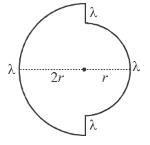Solution:

The electric field due to both straight wires shall cancel at common centre O.

The electric field due to large and smaller semi circular rings at O be E and E' respectively.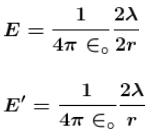∴ Magnitude of electric field at O is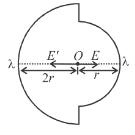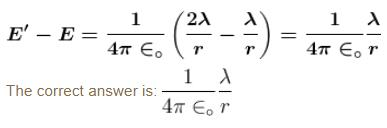QUESTION: 7

Two very large thin conducting plates having same cross sectional area are placed as shown in figure they are carrying charges Q and 3Q respectively. The variation of electric field as a function at  x (for x = 0 to x = 3d) will be best represented by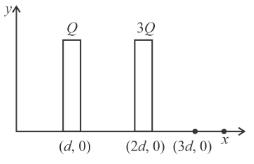Solution:

Using the formula for electric field produced by large sheet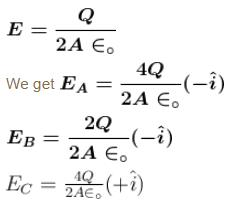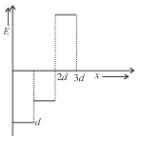QUESTION: 8

Three equal charges Q are placed at the three vertices of an equilateral triangle. What should be the value of a charge, then when placed at the centroid, reduces the interaction energy of the system is zero :

Solution: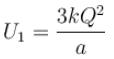where a = side of equilateral triangle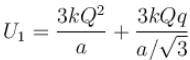where q is the charge brought at the centre

U1 = 0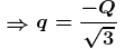The correct answer is: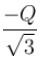QUESTION: 9

A point charge q moves from point P along the path PQRS (figure) in a uniform electric field E pointing parallel to the positive direction of the X-axis. The co-ordinates of points PQR and S are (ab, 0), (2a, 0, 0) (a, -b, 0), (0, 0, 0) respectively. The work done by the field in the above process is :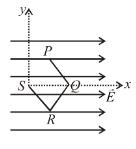Solution: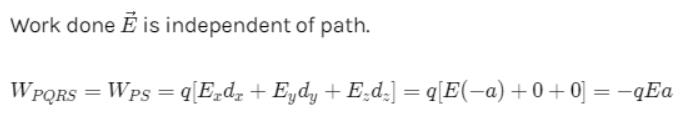Negative sign here shows that work has been done against feild.

QUESTION: 10

Four positive charges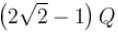are arranged at corner of a square. Another charge q is placed at the centre of the square. Resultant force acting on each corner is zero if q is :

Solution: